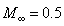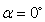Problem C1.4. Laminar Boundary Layer on a Flat Plate

Overview

This problem is aimed at testing high-order methods for viscous boundary layers, where highly clustered meshes are employed to resolve the steep velocity gradient. The drag coefficient will be computed, and compared with that obtained with lower order methods.

Governing Equations

The governing equation is the 2D Navier-Stokes equations with a constant ratio of specific heats of 1.4 and Prandtl number of 0.72. The dynamic viscosity is also a constant.

Flow Conditions, angle of attack, Reynolds number (based on the plate length) ReL = 1x106.

Geometry

The plate length L is assumed 1. The computational domain has two other length scales LH and LV, as shown in Figure 1.4. Participants should assess the influence of these length scales to the numerical results, and select large enough values that the numerical results are not affected by them.Figure 1.4. Computational Domain for the Flat Plate Boundary Layer

Boundary Conditions

As depicted in Figure 1.4.

Requirements

1.     A sensitivity study must be performed to find the appropriate domain size, whose effect on the drag coefficient is less than 0.01 counts, i.e., 1e-6.

2.     Start the simulation from a uniform free stream everywhere, and monitor the L2 norm of the density residual. Compute the work units needed to achieve a steady state. Compute the drag coefficient cd.

3.     Perform grid and order refinement studies to find a “converged” cd value with an error of 0.01 counts.

4.     Plot the cd error vs. work units for different h and p.

5.     Study the numerical order of accuracy according to cd error vs.1.     Submit two sets of data to the workshop contact for this case

a.      cd error vs. work units for different h and p

b.     cd error vsfor different h and p